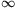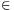Mathematical and Physical Journal
for High Schools
Issued by the MATFUND Foundation
 Already signed up? New to KöMaL?

#Problem A. 394. (February 2006)

A. 394. Let a1,a2,...,aN be nonnegative reals, not all 0. Prove that there exists a sequence 1=n0<n1<...<nk=N+1 of integers such that

n1an0+n2an1+...+nkank-1<3(a1+a2+...+aN).

(5 pont)

Deadline expired on March 16, 2006.

Solution. Define aN+2+aN+3=...=0 as well and look for an infinite sequence 1=n0<n1<... of integers for whichDefine the function f:[1,)R such that f(x)=f([x]).

The required sequence is constructed randomly. Take a random variable t[0,1] of uniform distribution. Set n0=1 andfor i=1,2,.... ThenThe value of n1 is 2,3,4 or 5 if,,, or, respectively. ThereforeandThere always exists a sequence 1=n0<n1<...<nk=N+1 such that

n1an0+n2an1+...+nkank-1<3a1+e(a2+a3+...+aN).

### Statistics:

 3 students sent a solution. 5 points: Erdélyi Márton, Paulin Roland. 0 point: 1 student.

Problems in Mathematics of KöMaL, February 2006